### Home > MC1 > Chapter 4 > Lesson 4.1.1 > Problem4-9

4-9.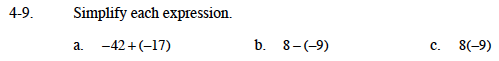For help with parts (a) and (b), refer to the Math Notes box from Lesson 3.4.4 below.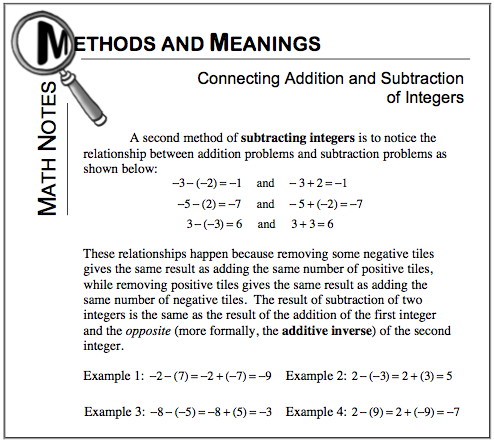Pay close attention to these similar examples. How can you use the examples to simplify the expressions?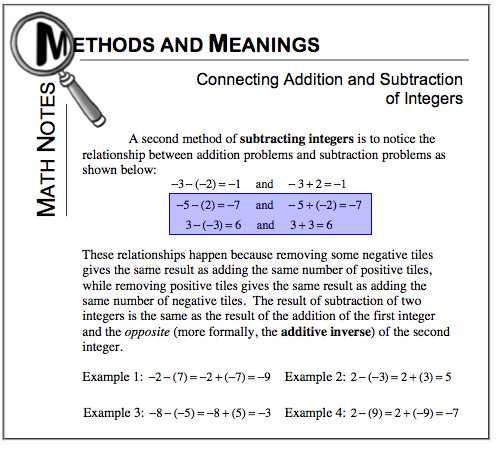Now, refer to the Math Notes box from Lesson 3.4.3 below for help with multiplication using negative numbers.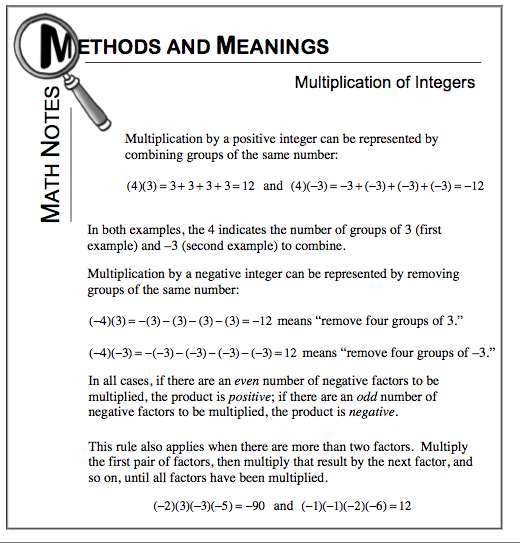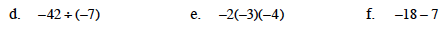Having trouble with division? Can you think of how this can relate to a multiplication problem?

Also, refer to problem MC1 3-127 in Lesson 3.4.4 for more guidance.

The expression simplified is 6.

Read the Math Notes box at the right for help with multiplying more than two factors.
This section may be especially helpful.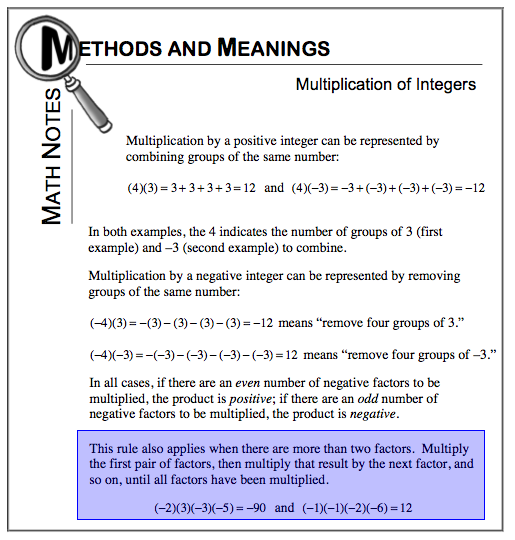The expression simplified is −24.

Subtracting is like taking steps backwards. Can you draw a picture to help solve this problem?

The expression simplified is −25.